Function Repository Resource:

LSystemPlot

Display an L-system

Contributed by: Robert Dickau
 ResourceFunction["LSystemPlot"][rules,axiom,n,delta] displays the L-string for the nth iteration of the list rules, starting with the string axiom after n iterations with an angle delta.

Details and Options

An L-system or Lindenmayer system is a parallel rewriting system that consists of a set of characters to produce strings and a list of production rules that replace each symbol from an initial "axiom" string a number of times. The L-system also needs a way to give the string a graphical meaning.
"F" is an instruction to draw a line segment one unit in the current direction. A plus sign (+) is an instruction to rotate the current direction one angular unit clockwise, and a minus sign (-) is an instruction to rotate the current direction one angular unit counterclockwise.
String replacements are made in parallel.

Examples

Basic Examples (4)

Four steps for Koch quadratic rules:

 In:=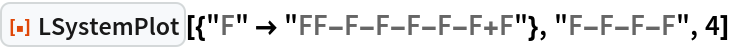Out=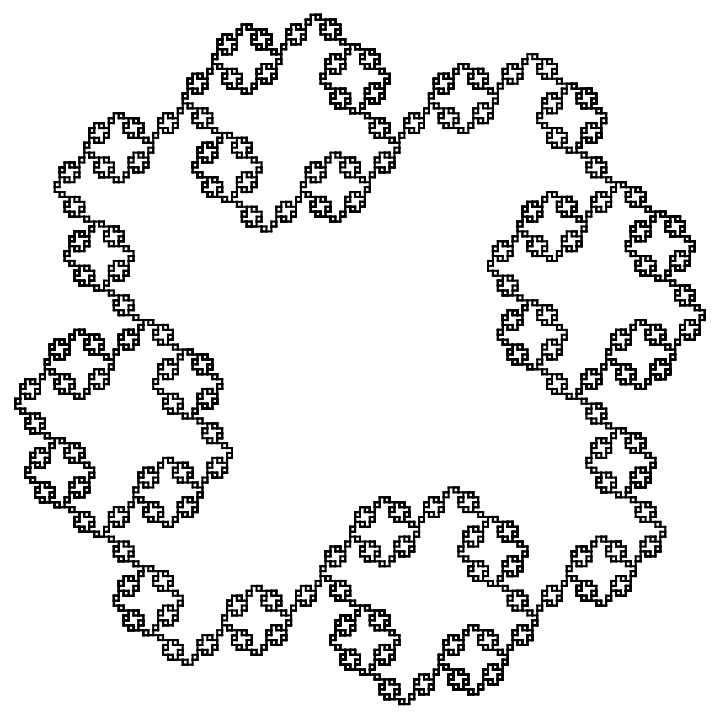Get just the resulting string after only one step:

 In:=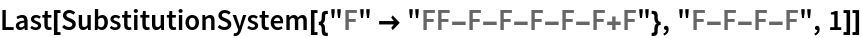Out=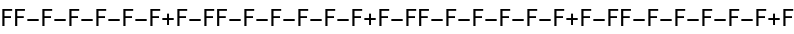Continuously modify the angle:

 In:=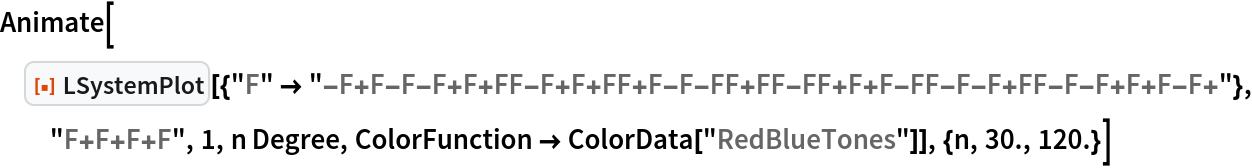Out=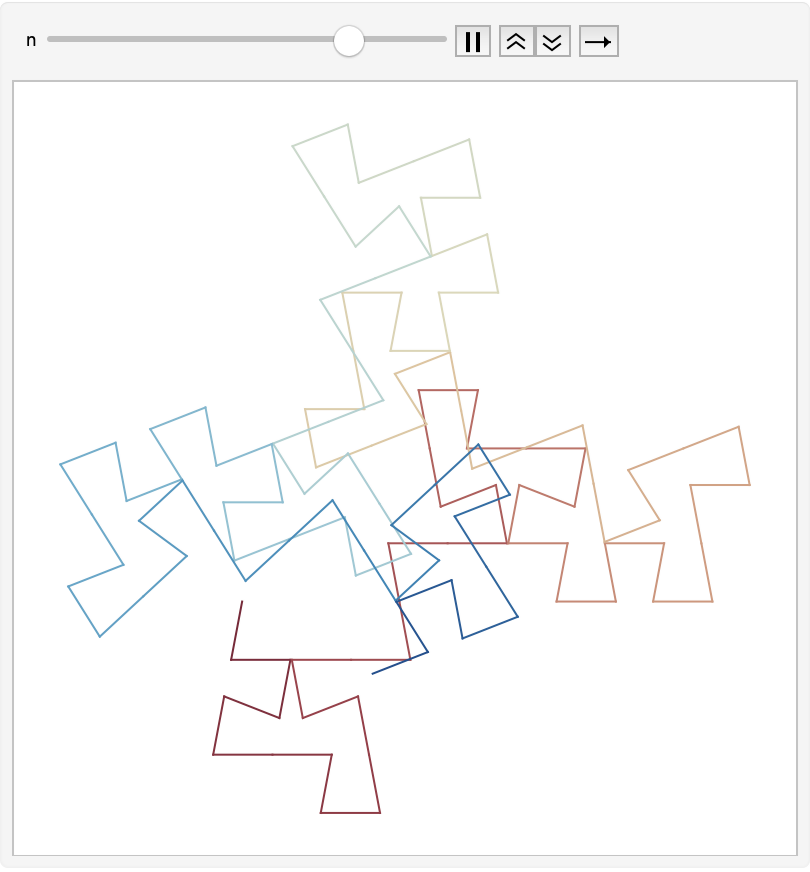Grid for named fractals:

 In:=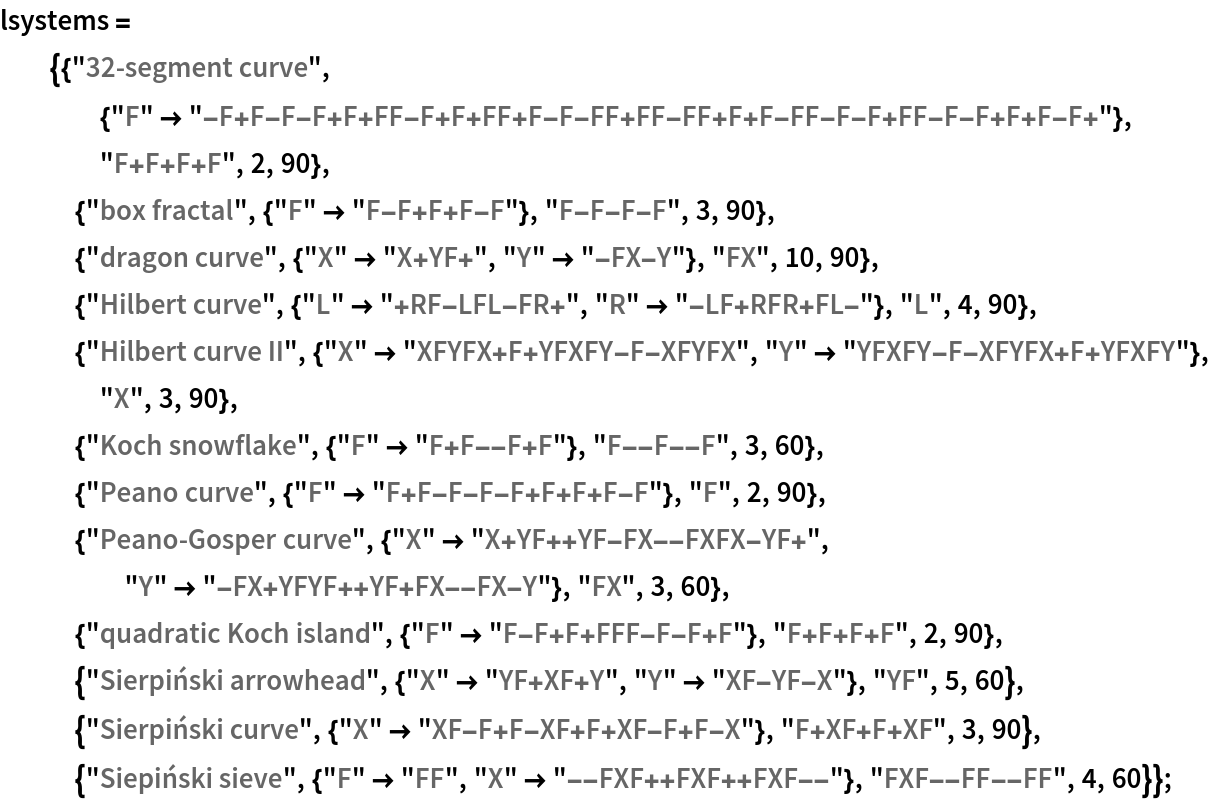In:=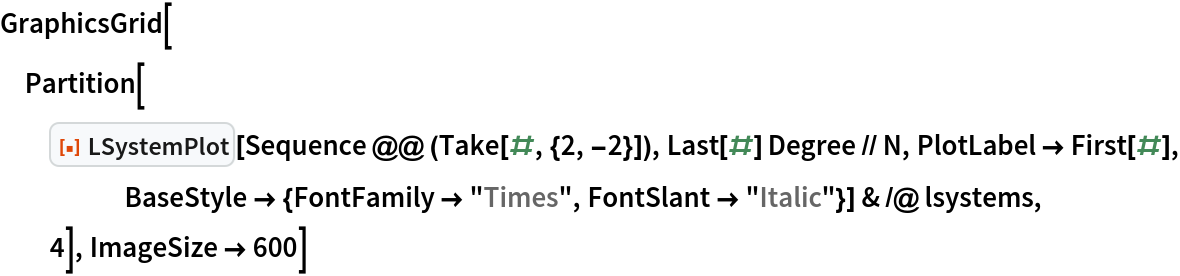Out=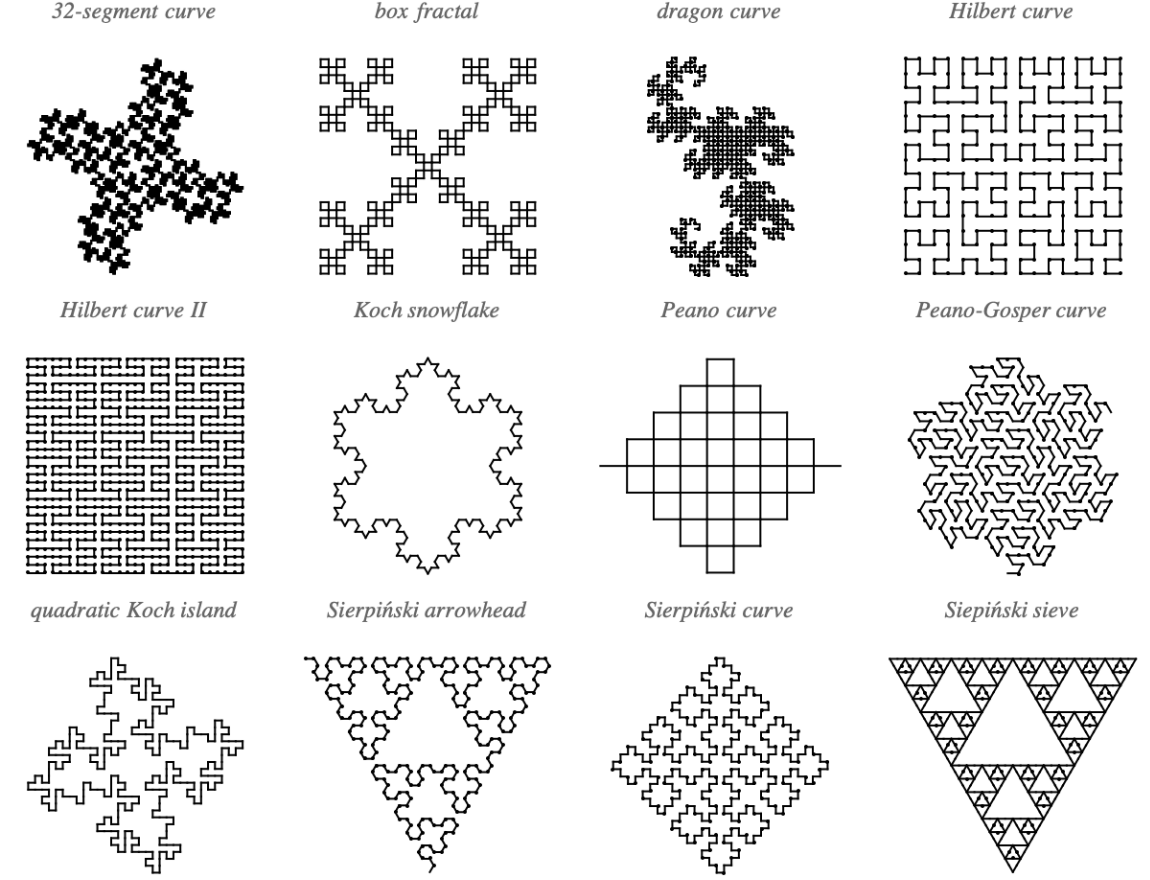Options (2)

ColorData (1)

 In:=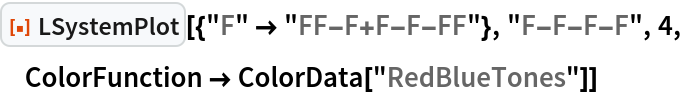Out=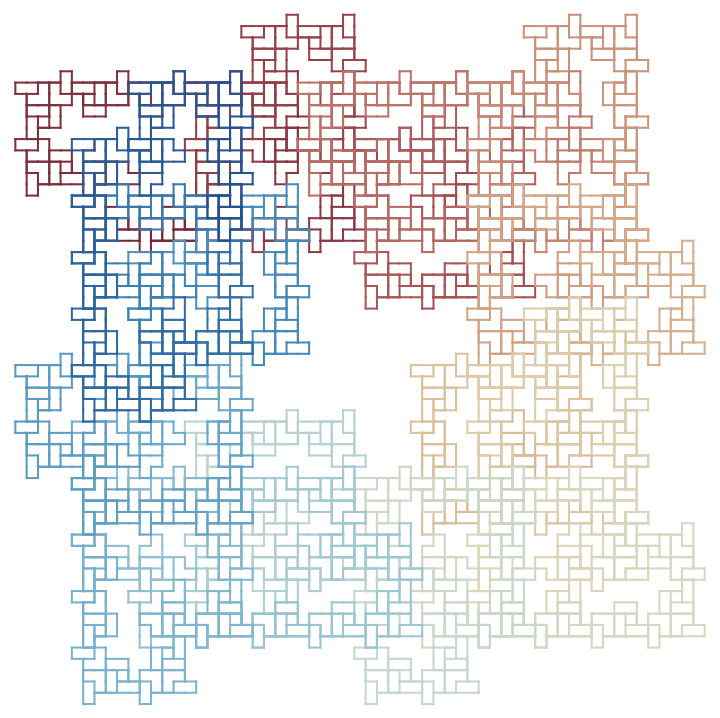PlotStyle (1)

 In:=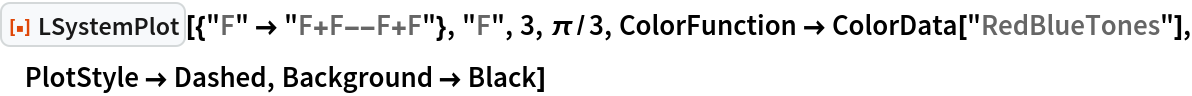Out=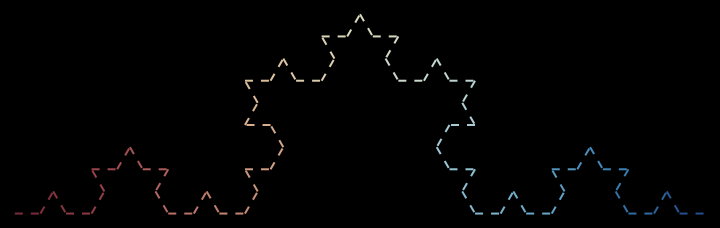Applications (3)

Convert to a BSplineCurve:

 In:=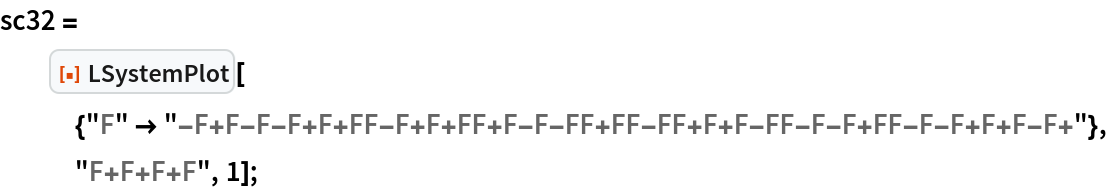In:=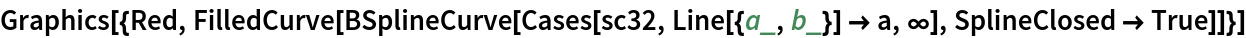Out=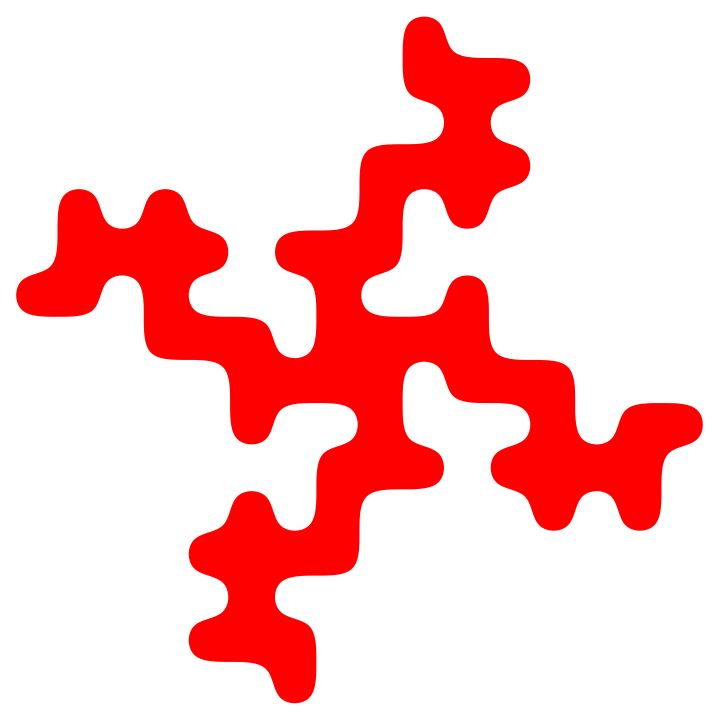In:=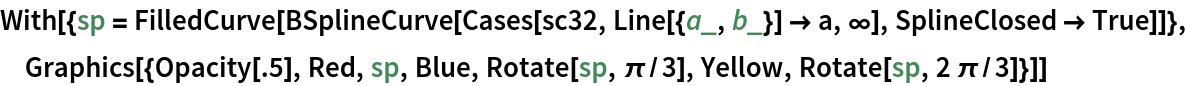Out=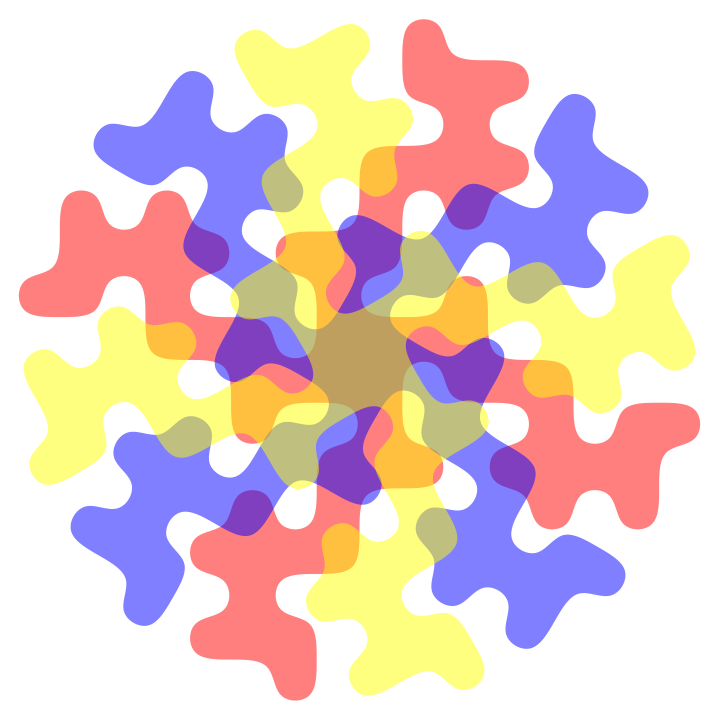Make a tessellation:

 In:=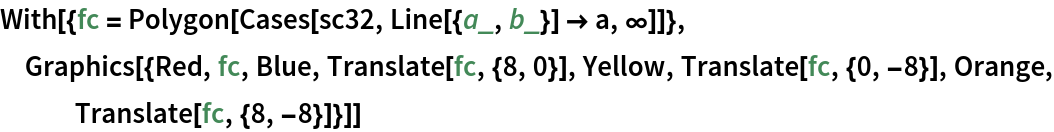Out=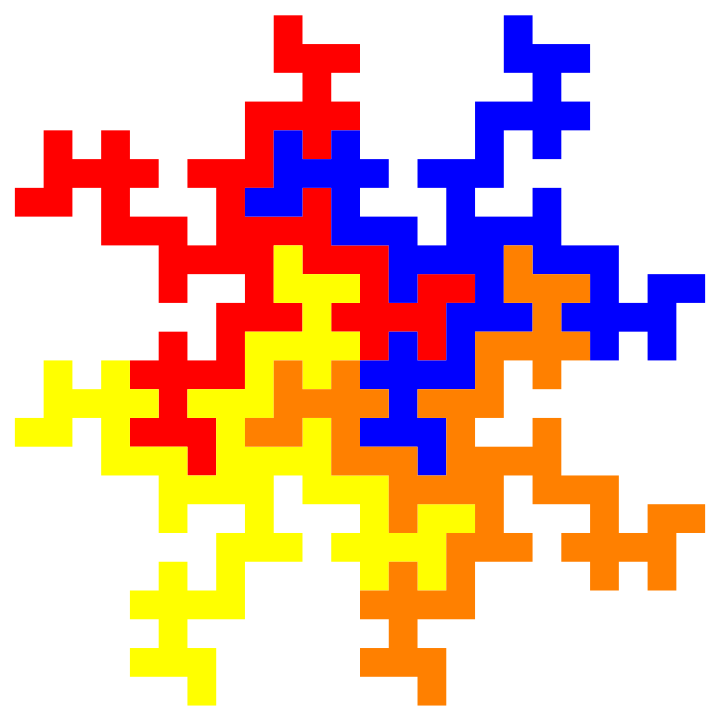Go to 3D:

 In:=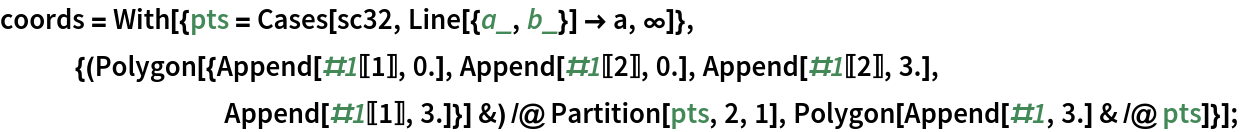In:=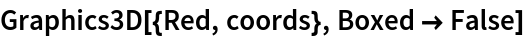Out=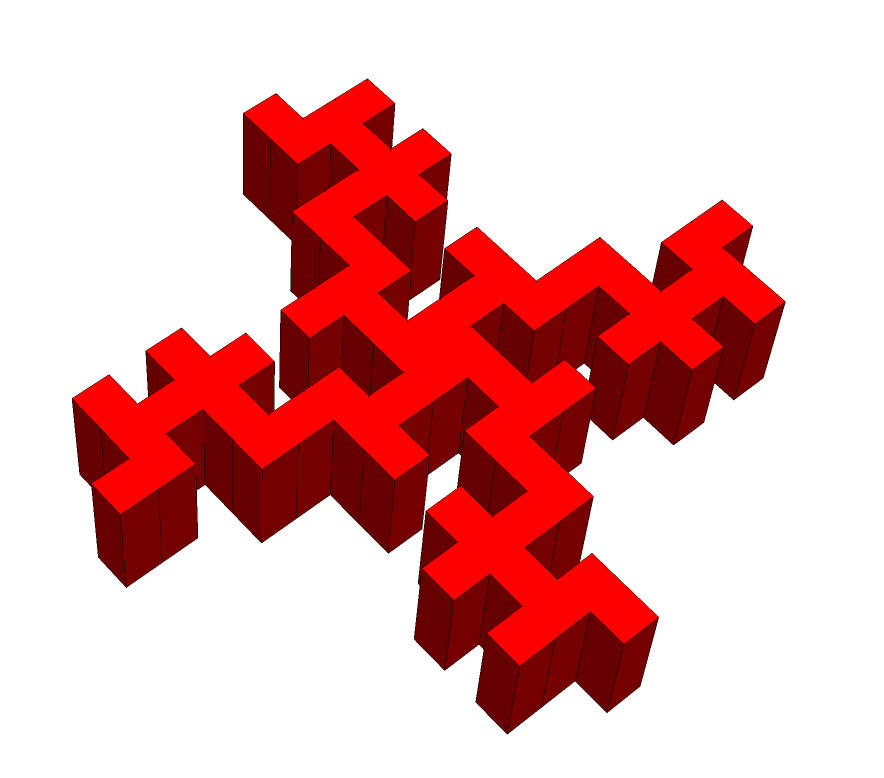Requirements

Wolfram Language 11.3 (March 2018) or above